# Stumped: How can I get a huge Earth-like planet?

I hope this isn't too broad.

I would like an Earth-like planet for November's NaNoWriMo that is just enormous, but plausible.

Then I started to get into material composition, proximity to the star, trying to achieve the right densities - well, you get the idea.

I'm trying to have 10x the radius of our current Earth, but with similar gravity (ugh) and at least somewhat similar climate and surface conditions; I do not need to have life on this planet, but minerals and liquid water are important to us. Not quite a Jupiter-sized planet, but much larger than ours. Other factors can be modified.

• Could you quantify "similar gravity"? – Dan Smolinske Apr 23 '15 at 18:11
• Within 0.75 to 1.25 if you need an exact range; thanks for asking. Happy to deviate, but I need to establish earthlike flora & fauna there (it doesn't necessarily have to have life on it already. – Mikey Apr 23 '15 at 18:51
• 10x radius gets you into the Gas giant range. Why not settle for something like 3x? – Serban Tanasa Apr 24 '15 at 12:34
• @theonlygusti yes I upvoted it, and it will most likely be the final answer. I'm hoping for a happy surprise answer that can make this planet believable. I also am happy with the "just enjoy writing it" responses. – Mikey Apr 25 '15 at 12:39
• Probably only works for certain types of story, but how about "smaller people"? Cf. Gordon Williams' "The Micronauts" for example. Or they could be engineered post-humans, or a long-lost colony that has evolved towards insular dwarfism, or etc. etc. – lessthanideal Dec 13 '15 at 2:28

Because you want a similar temperature and climate to Earth's, your new planet would have to be at approximately the same distance from its sun as Earth is, assuming both solar systems have similar-sized suns.

### For Earth's mean temperature of $15^{\circ}\mathrm C$, the planet would have to be about 1 AU from its sun.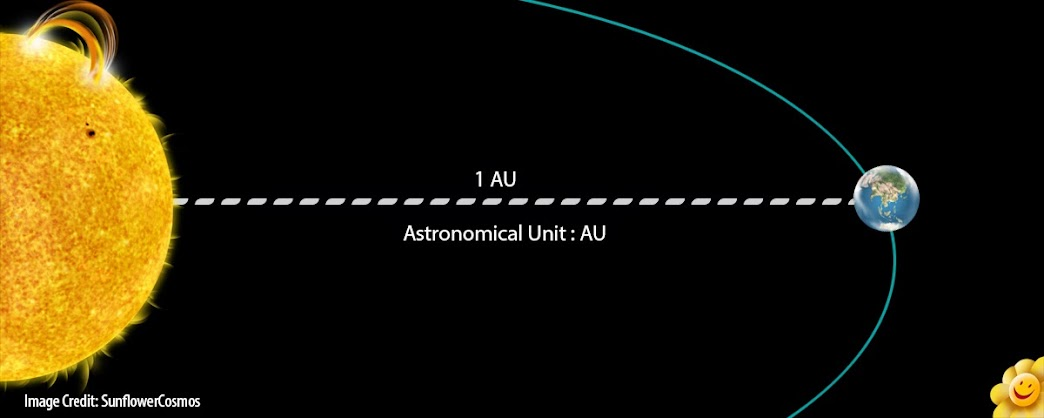For your planet to possess seasons and be similar in climate to Earth, it would need the same axial tilt, which is responsible for Earth's seasons. Earth's axial tilt is about $23.45$ degrees, your planet's would have to be similar for similar seasons.

Your planet would also need the same day-length so that each part of it will receive the same warming from the sun per day. If the day were longer, your planet might become a bit desert-like, very hot in the day, and freezing at night. That doesn't sound promising for life.

However, for a massive planet to possess a 24 hour day, the surface would have to be moving much, much faster than Earth's:

Earth's diameter is $12,756$ km, so has a radius of $6,378$ km.

Your new planet's radius is 10x larger, so would be $63,780$ km. That means your planet has a circumference of $2 \cdot 63,780\pi$ km, approximately equal to $400,742$ km.

For your planet to have a $24$ hour day, the surface would have to spin at $\frac{400742}{24}$ km/h, about $16,698$ km/h, which is pretty fast. (Earth's spin is only $1,673$ km/h.)

$$16,698~\mathrm{km/h}$$

which is pretty darn fast!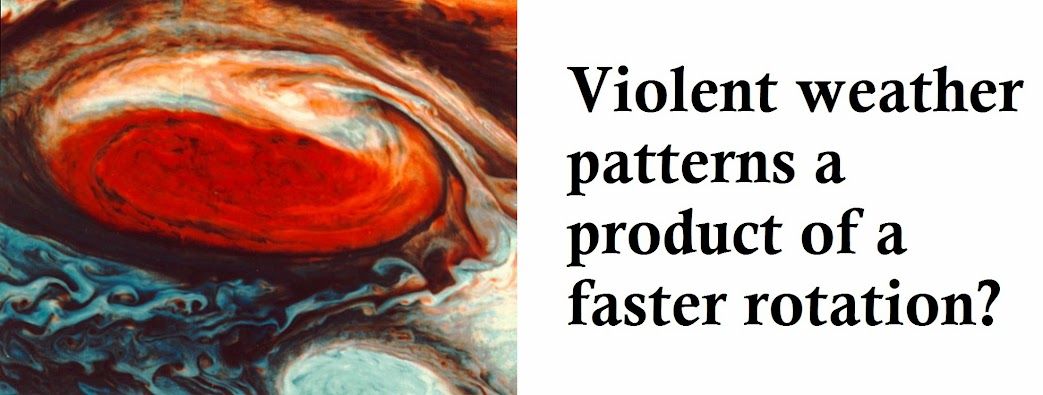Honestly, I was half-hoping that speed of this planet's rotation might be nearer Jupiter's $45,061$ km/h, so that I could talk about extreme weather patterns and phenomena such as the Great Red Spot.

Apparently your planet will not be subject to anything near as powerful as Jupiter's hurricanes, but your planet's spin is definitely fast enough to increase the strength of - and therefore devastation caused by - any of its storms.

Also, your planet's spin is still slow enough to lead to similar weather systems as on Earth, where winds are constrained to a hemisphere, and Jet Streams will become possible, which will help regulate your planet's climate and keep it more consistent with Earth's.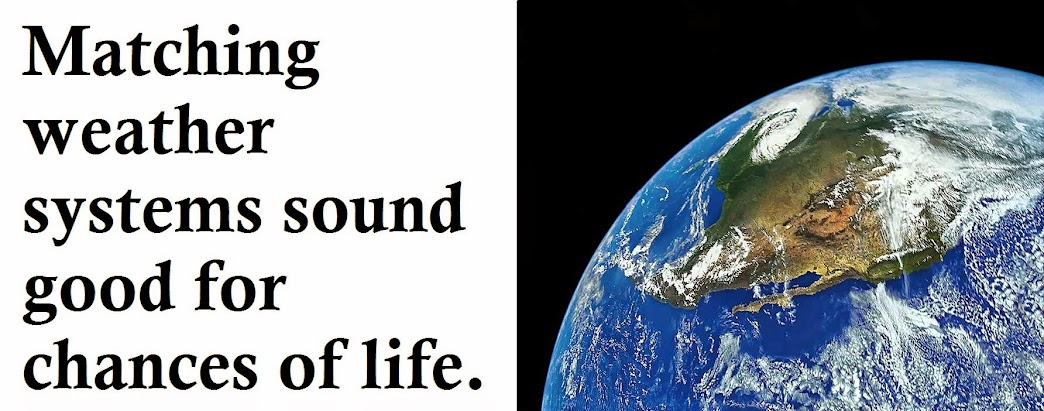However, we have yet to face the biggest problem imposed by a massive planet: keeping gravity somewhat similar to Earth's.

This is nigh impossible, as we will see after we calculate what density our planet would need:

Earth's density is $5,540$ kg/m3, and it's volume $1.08321×10^{21}$ m3.

Your planet's radius is 10x larger, therefore its volume must be 103x larger. This means that your planet's volume is $1.08321×10^{24}$ m3.

Usually, for two objects to have the same gravity, their masses must be the same, but the inverse square law also states gravitational attraction to be inversely proportional to the square of the distance between two objects.

Because your planet is 10x larger, anyone on the surface will be 10x further away from its center than they would be from Earth's center on its surface. Using this equality, we can calculate the necessary mass of your planet: $$\mathrm g = \frac{G\cdot M}{r^2}$$ Where $\mathrm g$ represents the accelration due to gravity ($\mathrm{m/s^2}$), $G$ the gravitational constant (6.673×10-11 N·(m/kg)2), $M$ the mass of our planet and $r$ the radius of our planet.

We can now rearrange and solve for $M$: $$M = \frac{\mathrm g r^2}{G} \\~\\ M = \frac{9.8 \times 63780000^2}{6.673 \times 10^{-11}}\\~\\ M \approx 5.974 \times 10^{26}$$

So, our planet's mass would have to be approximately $5.974 \times 10^{26}$ kg, which looks about right; Earth's mass is about $5.972 \times 10^{24}$ kg, and, for the same gravity, we'd expect our planet to be 100x heavier, which it is! Of course, we are suffering rounding inaccuracies, but so far, so good.

Now, we can calculate our planet's target density, using the equality that $$p = \mathrm{M/V}$$ where $p$ represents density, $\mathrm M$ mass and $\mathrm V$ volume, we see that: $$p = \frac{5.974×10^{26}}{1.08321×10^{24}}$$ so therefore the planet's density must be $551.5$ kg/m3 if you want the same attraction under gravity.

Saturn is the least-dense planet in our solar system, with a density of $687$ kg/m3. However, Saturn is a gas giant, composed mainly of Hydrogen and Helium: good luck mining for minerals in a cloud of Hydrogen!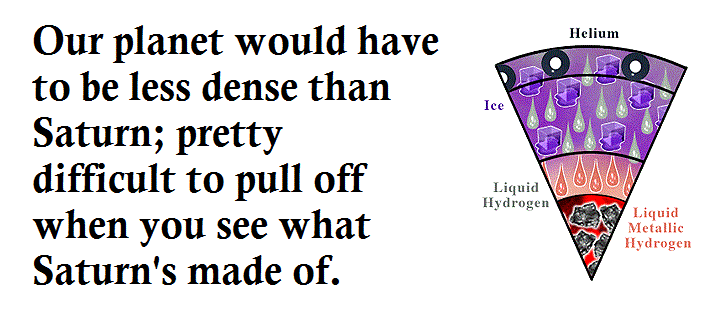### Your planet would need a density of $551.5$ kg/m3, 100 kg/m3 less than Saturn's!

So, it should be fairly obvious that you will never be able to get exactly the same surface gravity as you do on Earth, but fauna would be able to exist under different gravitational conditions, and, what's more, higher gravity means more pressure, which means minerals will form more readily!

There could be some special minerals which only form within the crust of this planet because of its strong gravity, making the planet more valuable!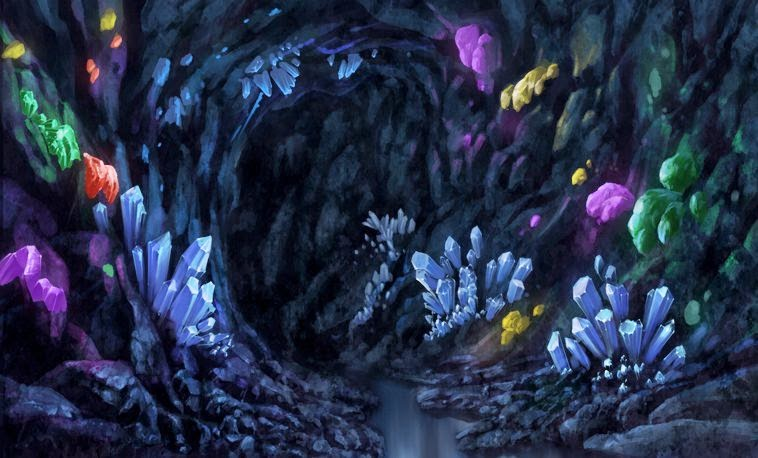However, to enable humans to land on this planet, it will be almost impossible to get the gravity weak enough (have you thought of using exoskeletons for manned missions on this planet?); the planet would have to be as sparse as possible.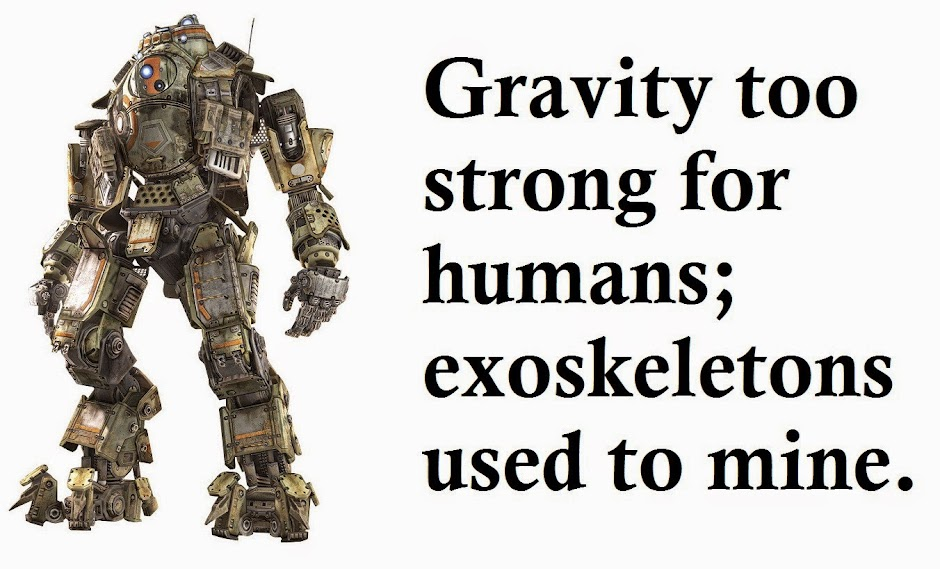If your planet was composed almost entirely of some really porous Vesicular rock, almost like a sponge, it would definitely keep down the density.

The same process which forms such rock could also create massive air-pockets, creating a cavernous planet with many underground tunnels and caves on the same scale as Erebor!

### A cavernous planet, made of porous rock, would remain reasonably sparse.

The crust of the planet should be as thick as possible, because a molten mantle and solid core would be more dense than this spacious, cavernous crust. This crust would also need constant renewal, so that collapses don't bring up the density, and to remain, again, more similar to earth.

However, tectonic activity would have to be minimal, to prevent metamorphic and denser igneous rocks being formed, so volcanoes would be necessary to keep rejuvenating the surface. Underground lava-flows might be commonplace, where it is still hot enough so that the lava cools slowly into some porous rock. Think about how awesome that could be; a massive, underground cave system where magma seeps up through the floors forming puddles of fiery lava!

The one thing we can't change is the presence of a solid, dense core; this is because a nickel/iron core is necessary for a magnetic field, which would protect the planet as the Earth is.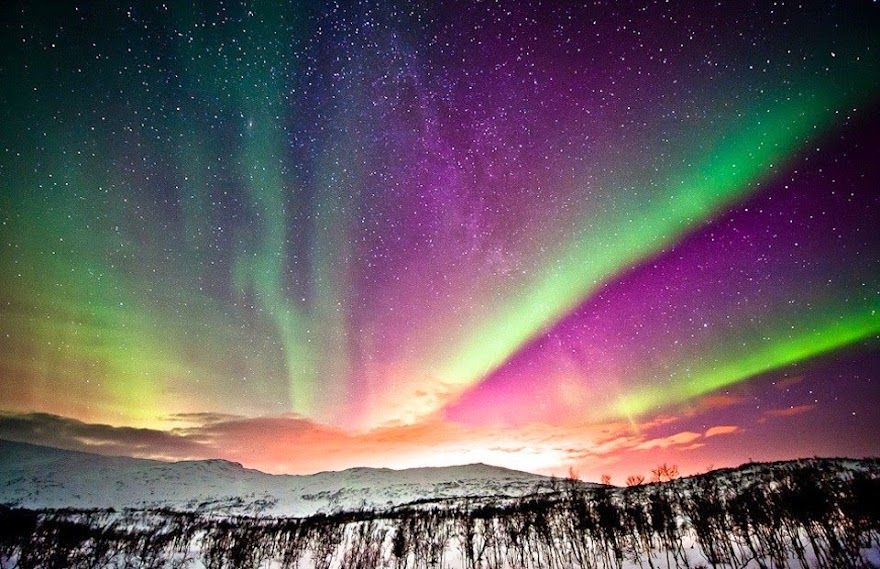The geomagnetic field would protect the planet from solar winds, thus retaining the Atmosphere and ozone layer, protecting inhabitants from radiation which would otherwise be harmful. Also, an atmosphere is generally a handy thing to have if you want life to live on a planet!

### A magnetic field is necessary to protect the planet like earth, so a dense core is also necessary.

We can actually calculate the density, and therefore acceleration due to gravity, of our planet:

Of course, our planet, possessing a molten mantle, liquid seas, and solid core, would likely be slightly more dense than Pumice (a Vesicular rock), but let's just say that Pumice is the most common rock on our planet, and everything else has a similar density.

The density of Pumice is $641 \mathrm{kg/m^3}$, so our planet's density would also be about $641 \mathrm{kg/m^3}$.

The volume of our planet is $\frac43 \pi r^3$, about $1.08321×10^{24}$ m3.

Now, using our planet's assumed density and volume, we can plug the values into our density equation: $$p = \mathrm{M/V} \\~\\ 641 = \frac{\mathrm M}{1.08321×10^{24}}$$ Rearranging, we get $$\mathrm M = 641 \times 1.08321×10^{24}$$ which is approximately equal to $6.94 \times 10^{26}$ kg. Our planet is pretty heavy!

Using this equation (same as before) $$\mathrm g = \frac{G\cdot M}{r^2}$$ we can solve for $\mathrm g$, the planet's gravitational acceleration: $$\mathrm g = \frac{(6.673 \times 10^{-11}) \times (6.94 \times 10^{26})}{63780000^2}$$ which is approximately $11.38 \mathrm{m/s^2}$.

Wait, only $11.38$? That's just $1.16$ Earths! I'm in luck!

Well, no, not really, not unless you make some other changes as well: the actual density of your planet would be much greater, as a large proportion of the planet would probably be magma (density: $3100$ kg/m3), and, if the planet is like Earth, a lot of the surface would have to be water (density: $1000$ kg/m3); the planet's mean density would obviously be above Pumice's $641$ kg/m3.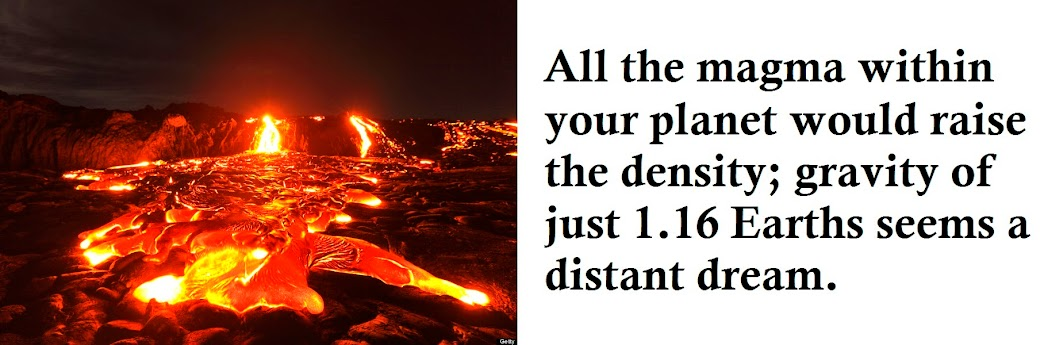However, if you discover some way of limiting the density of your planet's magma, this would no longer be an obstacle to your 1 Earth goal!

Maybe the magma is filled with air, think of carbonated water, a similar process could have trapped and compressed air within the magma of your planet.This idea also makes the Vesicular-rock-only surface more likely, as when the lava is released through fissures in the crust (think Volcanoes, etc.), the trapped air within the lava will expand, creating air bubbles. This is like getting the bends when re-surfacing from a deep dive: as pressure is released, compressed nitrogen within one's blood quickly expands into bubbles.

And my magma density figures were based on basalt; vesicular lava would have a lower density.

### Earth-like gravity still not too far-fetched an idea.

Anyway, that exoskeleton idea still seems pretty cool to me.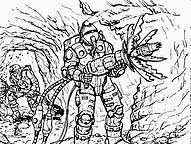• "Of course, our planet, possessing a molten mantle, liquid seas, and solid core, would likely be slightly more dense than Pumice (a Vesicular rock), but let's just say that Pumice is the most common rock on our planet, and everything else has a similar density." Is this a wise idea? Doesn't look like a minor difference to me… – o0'. Jun 25 '15 at 16:02
• @Lohoris No, it's not a wise idea, but writing an answer like this takes soooo much time; I spent at least 6 hours on it. Please, don't berate it too much :) – theonlygusti Jul 8 '15 at 20:43
• Hollows in the rock only work to a minor extent. Self gravity would crush out these holes aside from a very thin skin. The only way around it is to use actually lighter elements, which can only do so much. – Oldcat Dec 22 '15 at 18:19
• @Oldcat That's a very good point, but honestly, it is unlikely there will ever be a 10x Earth-like planet. Of course, the structural weaknesses could be compensated for by hexagonal or regular lattices of stone, as opposed to random arrangements of holes. Thanks for your comment though! I'm glad to know there's at least one person who's sifted through my entire answer ;) – theonlygusti Dec 22 '15 at 21:14
• Answers like this make me want to upvote them. Good job! – cst1992 Apr 19 '16 at 5:39

Basic Numbers
As it happens (and @TimB) pointed out, we have a planet that very nearly fits your preliminary description - Saturn.

Saturn's Stats

Diameter                             ~ 10 Earths
Mass                                 ~ 90 Earths
Density                              ~ 620 kg/m^3 - 6/10 as dense as water)
Gravity (@1 atm pressure region)     ~ 1.07
Temperature (@1 atm pressure region) ~ 134 K -> -139 C -> -165 F


In order to have a big planet with low gravity, you need a low density. Saturn has the lowest density of any planet in our solar system. It had to be made almost entirely of hydrogen to accomplish that. It means you'd have to fly to live on that planet.

You'd encounter no minerals, metals, few liquids, and certainly no bodies of liquid. In order to not be crushed in the depths of the atmosphere, you would need to fly to survive in Saturn's atmosphere.

Rotating bodies actually produce an apparent gravity that varies with latitude, as exemplified by the Earth's. On the Earth, this field varies by about 0.3% (~0.029 m/s) due to outward centripetal acceleration and 0.2% (~0.020 m/s) due to difference in the pole vs. equatorial radius. We can use this principal to imagine a planet that meets your needs.

Jupiter spins at a rate of 1 rotation per 10 hours. Its equator is about 7% larger than its polar circumference.

Planets become unstable and break apart at between 2 & 3 hours per rotation. At around this spin rate, the planet achieves a 100% oblateness (equator is twice as long as the polar circumference). The primordial Earth likely had this appearance shortly after the Theia Impact Event.

Acceleration due to gravity $$a_g = \frac{GM_p}{r^2}$$

Centripetal acceleration due to rotation $$a_r = \frac{v^2}{r}$$

Total acceleration is acceleration due to gravity minus centripetal acceleration: $$a_T = \frac{GM_p}{r^2} - \frac{v^2}{r}$$

$a_T = 10 \frac{m}{s^2}$
$G = 6.67 \times 10^{-11}$
$r = 10 \times r_{Earth} = 6.378 \times 10^7$ m
$v_{rotation} = \frac {C}{t} = \frac {2 \pi 6.378 \times 10^7 m}{2.5 hr \times 3600 \frac {s}{hr}} = \frac {4 \times 10^8 m}{9000 s}= 44527 \frac {m}{s}$

Solve for planet mass $$\left(10 + \frac{v^2}{r} \right) \times r^2 \div G = M_{planet}$$

Plug in numbers $$M_{planet} = \left(10 + \frac {50211396}{6.378 \times 10^7} \right ) \times 4.07 \times 10^{15} \div 6.67 \times 10^{-11}$$

$$M_{planet} = 2.49 \times 10^{27}$$ this is about 420x the mass of Earth. It is also about 1.25x mass of Jupiter.

If you approximate the volume of an oblate spheroid as $$\frac {4 \pi \left (\frac {r_{polar} + r_{equat}}{2}\right )^3 }{3}$$

then with a 2:1 equator:pole circumference, this body will have about $7.5^3 = 422$ times the volume of Earth. That gives this body a density of

$$\rho = \frac {2.49 \times 10^{27}}{4 \div 3 \pi r^3} \rightarrow \rho = \frac {2.49 \times 10^{27}}{1.09 \times 10^{24}} \rightarrow \rho = 2290 \frac {kg}{m^3}$$

This number is 3.5 times the density of Saturn (above) and almost twice that of Jupiter. This planet will be composed of hydrogen gas, water, and a rocky & iron core. It is unlikely to possess solid surface under conditions inhabitants could access them. It is possible that such a planet could have a liquid surface and would appear to simply be a giant water droplet the mass of Jupiter.

Other Fun Facts
Let's compare the acceleration due to gravity and centripetal motion:

$$a_g = \frac{MG}{r^2} \rightarrow a_g = 41 \frac{m}{s^2} = 4.2 g$$
$$a_r = \frac{v^2}{r} \rightarrow a_r = 31 \frac{m}{s^2} = 3.2 g$$

Centripetal acceleration helps, however, increasing the equatorial radius helps much more.

$$a_{g at pole} = \frac{MG}{r^2} \rightarrow a_g = 163 \frac{m}{s^2} = 16.6 g$$

That's 16.6 $g$ at the poles while you only feel 1 $g$ at the equator. As a rough approximation, your distance between equator and pole with vary according to the sine of the latitude like this:

$$g_{apparent} = 1 g + sin \left( latitude \right) \times 15.6 g$$

Other reference information
If you've got the time and interest, this video:

The Surprising State of the Earth after the Moon-Forming Giant Impact

provides a lot of information about high spin rate planetary bodies, how they get that way, some simulations, and other information pertinent to your interests. It runs about 1 hour long but I enjoyed it very much and found it highly informative.

• I'm still not convinced by the varying surface gravity - physics.stackexchange.com/questions/178055/… – Tim B Apr 24 '15 at 8:08
• May I ask why in the centripetal acceleration you are dividing my 3600 seconds/hour instead of multiplying? In my opinion, that answer is wrong by a factor of about 10,000,000 – Serban Tanasa Apr 24 '15 at 12:39
• Downvoted for atrocious physics – Aaru Apr 24 '15 at 17:46
• @SerbanTanasa $\frac{num1}{\frac{num2}{num3}} = \frac {num1 * num3}{num2}$. So as you suggest, I am indeed multiplying by 3600. If my mass number is 10,000,000x too low, then you're implying the mass of the hypothetical planet would be 10,000,000x of Jupiter, a mass of $2.5 \times 10^{34} kg$ (and have an apparent surface gravity of Jupiter). Whereas at 100x of Jupiter we get fusion in the core, at 1200x of Jupiter we get the Sun's mass, and 120,000x of Jupiter is the mass of the most massive known star. What planet is 100x the most massive known star? – Jim2B May 6 '15 at 1:51
• @Aaru, if you're aware of any error in the physics, please point it out. – Jim2B May 6 '15 at 1:58

Let me say it outright from the start: you can't get a $10 \times R_\oplus$ radius world to be inhabitable by anything resembling humans.

Before you despair, let me remind you that planetary surface increases with the square of the radius, and Earth is huge to begin with: 510 million square kilometers. So for twice the radius, you get 2.04 billion square km, and for three times the radius, you get 4.5 billion square km, nearly 10 times larger!

Imagine how different history would have been had the Earth been that large. The larger surface area might mean slower days with longer nights, or perhaps faster orbital rotation. The terror of the night would be prolonged, or storms might be more massive than anything we know here on Earth. The vast oceans would regulate and moderate climate more than on Earth with their vast heat capacity, while waves might build up over the ocean vasts to truly astonishing heights (100m or more). The atmosphere itself would likely be deeper and denser, and given the higher planetary mass, retain more of the primordial gases.

So how do you get such a thing? The most likely process would be to start from a Chthonian planet, have it drift away from the star and rebuild some of the atmosphere from comets and eruptions.

• You say a Chthonian planet why? I'm guessing that it's less likely to accrete rock only, so you get rid of the gas later? Maybe it forms close to the star and/or is a merger of multiple rocky planets. – JDługosz May 9 '15 at 20:58
• Why would a 10 time radius world be inhabitable by hmans per se? To have similar gravitational pull on the surface the material of the planet has to one tenth of density of the material of the earth. If tilt, solar radiation, spin, magnetic field and so on is comparable, I see no real reason why it must be inhabitable. – Mnementh Jun 10 '15 at 12:00

For temperature and suchlike requirements you just need to orbit at roughly the same distance from the star.

The tricky thing here is the gravity, to have 10 times the radius then you either need to make the planet from something much much less dense than earth (which is improbable) or you need to spin the planet really fast.

If you spin the planet then it will flatten out into more of a disk shape but the equator could be ten times the radius of earth's and you could still get earth-level gravitational forces everywhere on the planet.

The planet would be the same "height" (pole to pole) as earth but much wider.

You'd get some very strong weather and Coriolis effects but the apparent gravity would be just fine.

• Note that spinning doesn't result in constant apparent gravity on the surface. Saturn's equator vs poles differ by well over 10% because of its high spin. Spinning still decreases averaged gravity, so it still solves the problem, but extreme uses of it will result in extremely non-constant gravity. – AlanSE Apr 23 '15 at 16:35
• @AlanSE Actually I'm not sure about that. The planet will tend to flatten out to give constant gravity at the crust - since that's the equilibrium point. I've been trying to work it out in my head and that's the only result I get to. – Tim B Apr 23 '15 at 17:40
• @AlanSE Although the Saturn case is interesting, see here: physics.stackexchange.com/questions/66140/… – Tim B Apr 23 '15 at 17:42
• I totally see where you're coming from, because I went down that path myself at first. If you consider the combined gravity + rotational apparent field (sometimes called a "false" field), then the critical insight is that the surface is defined to follow orthogonality - the fundamental physical condition is that we feel gravity at a right angle to the ground. That also makes it an equipotential line. Just like following a line on a topology map (in analogy to vector fields), the mountain's slope could be constant over the line, or it could not be. The fictional planet Mesklin had it right. – AlanSE Apr 23 '15 at 18:00
• @TimB - See Hal Clement's "Mission of Gravity" about a planet where gravity varies from 3 g at the equator to 600 g near the poles. – Oldcat Apr 23 '15 at 18:37

Ignore a little bit of science.

In reality, worlds that big have more gravity than Earth. All of the things that have a surface area many times that of Earth, are solid, and have the same gravity, don't occur in nature, and often have significant structural flaws requiring construction of unobtaium in order to remain in one piece. All of that doesn't mean that you can't write a great story taking place on one. Niven's Ringworld is one such story that takes place on something that probably couldn't exist, and relies on some unobtanium wires to hold things together, but is still widely enjoyed and admired.

Your story, for example, could take place on a world that is hollow. A hollow world could quite easily fit all of the criteria that you want, provided that you ignore the fact that hollow worlds can't actually form. You can explain this away with 'aliens made it with super advanced technology,' or 'magic', depending on what type of story you're trying to tell. If you're really feeling creative: aliens created it with magic. Alternately, you don't have to explain it at all. You can have enjoy writing about it and others can have fun reading about it without knowing exactly why or how the world is hollow.

Have fun writing!

• What if alien miners removed the planet's core? Nickel, iron and especially iridium are all damned handy. My point here is I can think of a good reason to remove the dense planetary core. – Peter Wone Apr 23 '15 at 22:21
• There's a good reason to mine one, but the planet would collapse inward under the force of gravity once the core was removed, unless the aliens use some advanced technology to keep that space open. I don't think we know of a way to do that, so some science will need to be ignored for them to be able keep the planet hollow. – ckersch Apr 23 '15 at 22:43
• And, if the core was removed, no more geomagnetic field; everything on the planet would die. – theonlygusti Apr 25 '15 at 13:35

The real SFNal way to do this is simply to "pave over" Saturn. A "supra-mundane terraformed world of Saturn" essentially has the atmosphere of Saturn covered over with some material which provides a solid "floor" for people to sand and work on, support buildings and so on.

Materials like graphene or a "fabric" woven from Fullerine cables will provide the high strength to weight ratio needed to make this plausible, and while it may seem a bit like standing and working on a trampoline, the reality is the structure will be so vast that most flexing will be damped out and you and I would hardly feel anything.

Now the questions of atmosphere and climate will be more difficult to answer. An atmosphere can certainly be added to the outside of the supra-mundane world of Saturn, importing materials from the many moons of Saturn and importing extra nitrogen from the distant moons of Neptune and bodies from the Kuiper belt (if you can cover over Saturn, you can easily import materials from deep space), as well as oceans and soil to support whatever sorts of biospheres you desire. Since you are so far from the Sun, a system of mirrors will be needed in orbit to provide extra illumination.

Weather and climate will be very different from that of Earth simply due to the effects of scale, and there will also be lots of variables based on how you compose the atmosphere, the percentage of land to ocean you choose to create and the details of how and where the platoons of mirrors orbit and illuminate the planet, so there will be a lot of handwaving that can be done to meet your needs as a story teller. I would expect that the early period where the atmosphere and biologicals are being added will be marked by violent discontinuities as equilibriums are established and then disrupted, so people living there might not settle on the supra-mundane world unit late in the building and seeding process.

Actively moving Saturn into a closer orbit around the Sun is also possible, but the amount of time and energy needed is "astronomical". If a sufficient number of asteroids, comets or similar sized bodies could be sent in looping orbits from the Kruiper belt to pass by Saturn, the planet could transfer some of its orbital energy to these bodies (speeding them up and flinging them from the Solar System) and gradually moving into a closer orbit around the Sun. This will require a lot of planning, as these bodies could crash into planets or spacecraft in the outer system, while as Saturn approaches Jupiter orbit, unexpected effects might arise out of gravitational interactions, resonance orbits between Saturn and the Asteroid belt and even the inner planets. It might be better to stick with mirrors.

So maybe our descendants in AD 2500 might be starting a project of this magnitude, it will certainly need a vast amount of resources and the ability to control vast amounts of matter and energy in order to do so.

• Moving Saturn might reduce its gas content, if it was done by ejecting material. – JDługosz May 9 '15 at 20:49

An interesting idea would be to have a hollow planet... An old dr.who episode features a pretty awesome plot, where the villains control a giant pirate ship planet that is hollow and can mine other planets by teleporting around them

• To keep an earth-mass or greater planet hollow, you need unreasonably strong materials, or it would just collapse into a small ball. – Oldcat Apr 24 '15 at 18:53
• @Oldcat Yeah, I'm getting that if a structure as described in the question was made of diamond, its compressive strength would be overloaded by a factor of about 150. – alessandro Apr 25 '15 at 21:09

Planets don't get much bigger than Jupiter: add more mass and they get denser in proportion, keeping the same radius all the way into brown-dwarf territory.

Jupiter is 11 Earth radii, but only 320 Earth masses. 10× across means 1000× the mass.

So why can't you pile up that much rock? Common planetary formation seems to not do that, from the sample we have thus far. But the Universe is large, making improbable things likely somewhere. You need a way to collect rock without snowballing up all the ice and gas once it gets sizable. Rocky planets form inside the "frost line", but they run out of material.

Here's some random ideas: a very large and dusty source cloud forms a blur hypergiant and has lots more "nearby" inside the frost line. Furthermore once the star ignited it ionized and blew off the leftover gas leaving dust around longer. More dust arrives from farther regions that are disturbed by other migrating planets and fling half of it inward.

The merger of the inner rocky planets doesn't result in the star swollowing it but it's close: it's flung out of the system, and is captured by another star in the same nursery, before the cluster dispurses, so it's not too outrageous to be captured and ot remain rogue.

Tidal forces of the close approach can rip off the gas amd ice leaving even more pure rock, which can be topped off after arriving at the new star.

You could have a hiarchial binary system with exotic members, like a neutron star. There are pulsar planets...where did they come from? Maybe reformed after the supernova or Chthonian processes. With the long-term instability and past near approaches of the binary suns to the binary exotics, planets may easily get shuffled.

A pulsar planet combines with a mundane terestreal planet and icy bodies to form an outer covering of rock, and replaces the terestreal planet around the sunlike star.

The believability devises are:

• if you want a rare outcome, do a lot of shuffling!
• site processes we know about but don't understand yet.
• introduce a spectrum of (currently unknown) systems so this is not a total outlier.

This Equation will help you understand why the Earth can not be much larger than it is now and still allow life to stay alive. The upper limit is around 7000 km radius, and even that might be too big as the surface gravity will compress the atmosphere too much, and raise the surface temperature above 150 F. The Rocky Planet Density Equation :

Density = (1+Pi) x 10^-1 * R^3 + (1+sqrt 2) x 10^-1 * R + 2900 kg/m^3.

The best fit is R = 6372.4567 Km producing g = 9.815 m/sec^2 surface gravity. A 7000 km radius produce a 1.19822 g surface gravity.

## A cache of negative matter

Everything is simple except for making a giant planet with similar gravity to Earth's... so add some unobtanium, which conveniently explains why the planet's minerals are such a valuable resource.

Perhaps the planet has a cache of negative matter inside it. This is not antimatter, but a hypothetical form of exotic matter that has negative gravity, which repels mass instead of attracting it. Such material is necessary for stable wormholes and one possible FTL warp drive, so if those exist in your universe I would imagine negative matter to also exist, and moreover be very valuable.

There are no known particles with negative mass, but they are theoretically possible according to the current understanding of physics.

Your planet would have to be almost exactly half normal matter and half negative matter, with only an Earth's-worth of regular matter more. However, it should be reasonably stable; negative matter is still attracted by normal gravity, so you don't have to worry about the planet spontaneously exploding or negative crystals falling into the sky as soon as they are mined.

Magnetism is "stronger" than gravity, right?

So what about an artifical planet with layers of nanite-controled magnetized iron (combined with sort of forcefield) that holds thicker layers of rock, many with air and water on them? The planet is divided like an onion or a spherical sky scraper, many layers have life, perhapse from different planets. Carefull use of nanomachines is how the magnetized mega-structures were made in the first place.